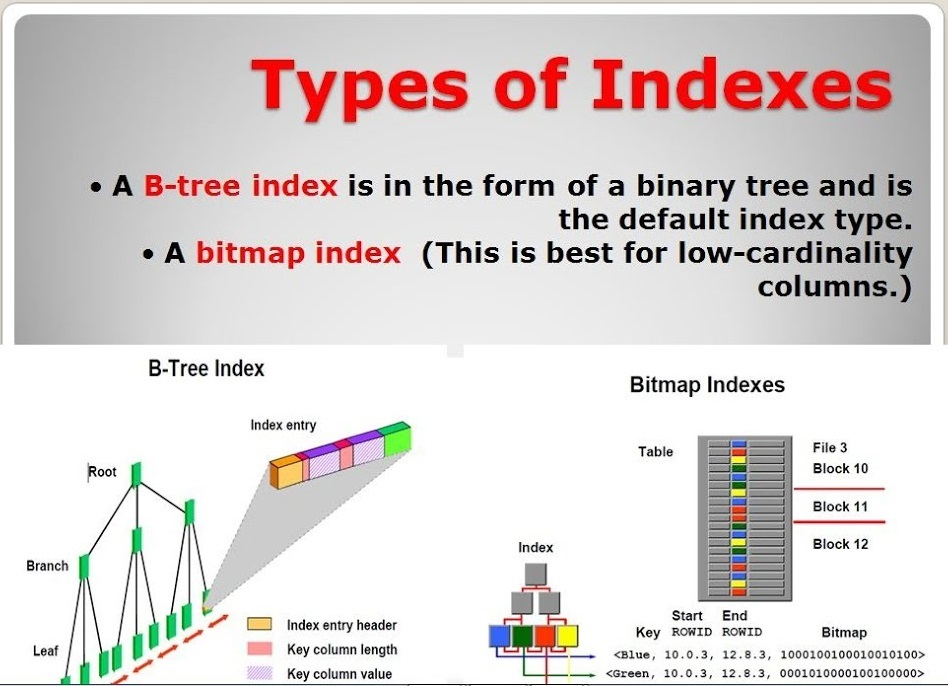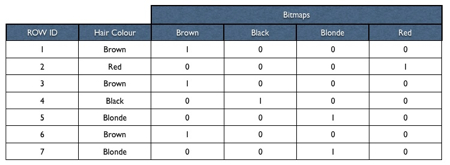# Index Types and Index Create in Oracle Database

I will explain Index Types and Index Create in Oracle Database in this article.## Index Types and Index Create in Oracle Database

If you don’t know What is the Index and Advantage or disadvantage of Index, you should read following article before this.

What is an Index ( Advantages and Disadvantages ) in Oracle Database

### Index Types in Oracle Database

There are 2 types of index types that are commonly used in Oracle Database as follows.

1. B-Tree Index ( Balanced Tree Index )
2. Bitmap Index

1- B-Tree Index ( Balanced Tree Index ): This is the most widely used index type and is the default index in Oracle database. The structure of B-Tree Index for a set of characters is as follows.B-Tree Index starts with the outermost Root block when Oracle accesses the data. Then goes to the appropriate Branch in the second leaf ( Second level ) and then goes to Last Leaf Blocks and reads the ROWID information found in this leaf. Oracle finally reads the requested block using the ROWID information.

For example, when searching for the name Luis in the Tree above, first go to ROOT, then to Related BRANCH (LU Branch)  and finally to the correct LEAF and find the correct record.

### Index Create in Oracle Database

Likewise, the structure of the B-Tree Index for a sample record set consisting of numbers is as follows.You can create B Tree index like following.

```SQL> create index INDEX_NAME on TABLE_NAME (related_column);
SQL> create index MID_IX on customer(customer_id);
SQL> create index FID_IX on invoice(invoice_id);

```

You can specify and create index storage and other attributes like following.

```CREATE INDEX MEHMET.ACCOUNT_IX ON MEHMET.ACCOUNT
(ACCOUNT_ID)
LOGGING
TABLESPACE DATA
STORAGE    (
BUFFER_POOL      DEFAULT
FLASH_CACHE      DEFAULT
CELL_FLASH_CACHE DEFAULT
)
NOPARALLEL;```

2. Bitmap Index:  This index type is the most commonly used index type after B-Tree. This type of index is used when certain values repeat continuously. For example, there are only 2 values for the Gender Column. This value is repeated for each user, male and female, or columns where a limited number of values can be entered, such as Yes or No. What we call a bitmap is a bit map and consists of 0 – 1 s. The bitmap index holds the ROWID of each record and the bitmap value. An example recordset showing the structure of the bitmap index is as follows.You can create the bitmap index as follows.

```SQL> create bitmap index INDEX_ADI on INDEX_ATILACAK_TABLONUN_ADI(ilgili Kolon(lar));
SQL> create bitmap index CIN_IX on musteri(cinsiyet);```

Apart from these indexes, there are indexes created with similar logic as follows.

• Function-Based Index:  In this type of index, When you use the function in the Where condition of your queries, it will not use the index unless you have created the index with this function. In this case you need to create Function based index . An example of this and the corresponding script is as follows.

`SQL> Create index MAD_IX on customer(name);`

When creating an index as above, the following query will use the Index.

```SQL> select * from customer where lower(name)='MEHMET';

```

In this case you need to create Function based index as follows.

```SQL>  Create index MAD_IX on customer (lower(name));

```

When you create an Function based index like above, the query will use the index despite the lower function put in the where condition.

• Concatenated Index: This type of index is actually the advanced version of the B-Tree index. With Concatenated Index we can concatenate more than one column in an index. For example: In the Customer Table, we can index and query the Name and Surname column.

```SQL> Create index MAD_IX on customer(name,lastname);

```

When creating an index as above, the following query will be able to use the index.

```SQL> select * from customer where name='mehmet' and lastname='deveci';

```
• Unique Index:  This type of index is the advanced version of the B-Tree index structure. Normally, the B-Tree index structure is Non-Unique. When we use the Unique keyword to create this index type, Oracle will create a Unique index. This type of index is especially used on columns with unique records. It is created as follows.

```SQL> Create unique index MTC_IX on customer(SSID);

```
• Reverse Key Index:  This index is an improved type of B-Tree index. In this index type, the value of the index key is always reversed. Ex; Suppose that our index values are 123, 456, 789, in this case Reversed key index values will be 321, 654, 987. This index is created as follows.

`SQL> create Index MID_IX on customer(customer_id) reverse;`

Read the following post if you want to learn what is the Index and Why Index ?

What is Index and Why Should We Use Index?

##### Do you want to learn Oracle Database Performance Tuning detailed, then Click this link.

4,739 views last month,  1 views today